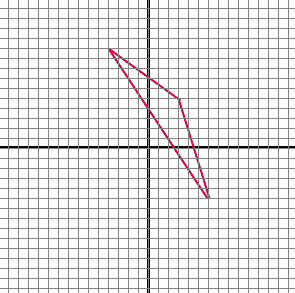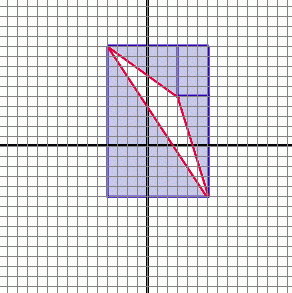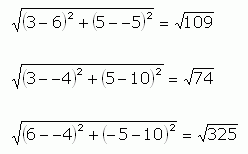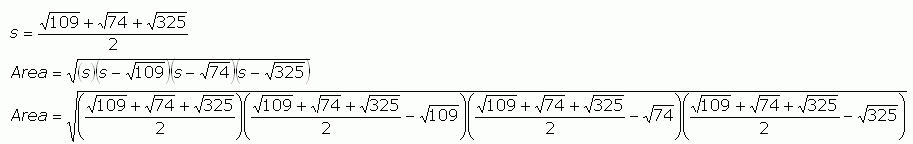SEARCH HOMEMath Central Quandaries & QueriesMy question is 'find the are of a triangle whose vertices have coordinates (3,5),(6,-5), and (-4,10) Please help. Thank you, BettyHi Betty. There are a couple of ways to do this.One way is to divide it into right triangles and rectangles and add or subtract areas as you need to. This is easy to do, but rather tedious:So the area of the red triangle is the area of the big rectangle (10 x 15) minus the area of the small rectangle (3 x 5) minus the areas of the three right triangles (½ x 7 x 5, ½ x 3 x 10, ½ x 10 x 15)

Another way to solve it is by algebra: Find the lengths of the sides using the Distance Formula, then use Heron's Formula to find the area of a triangle given its three side lengths.

Distance Formula calculations:Heron's Formula calculations:I presume these two approaches will give the same answer (they must!) but you can decide for yourself which approach is easier!

Cheers,
Stephen La Rocque.Math Central is supported by the University of Regina and The Pacific Institute for the Mathematical Sciences.